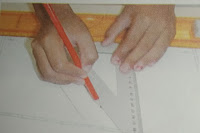# Lesson Planning of Parallel and Non-Parallel Lines

Lesson Planning of Parallel and Non-Parallel Lines

Subject Mathematics

Students` Learning Outcomes

• Recognize parallel and non-parallel lines.
• Draw a parallel line to a given straight line using set-square.
• Draw a line which passes through a given point and is parallel to a given line

Information for Teachers

• Lines that lie in the same plane and don`t meet one another are said to be parallel lines.
• During this lesson teacher should consult textbook wherein and whenever it is required.

Material / Resources

Writing board, chalk/marker, duster, straws, pebbles, geometry box, textbook

Introduction

• Recall the concept of line and tell that there are some lines which never meet and never touch each other. They are called parallel lines. When curiosity rose present the following:
• Take two straws and place them in position to show straight parallel lines.
• Take some pebbles and arrange them on floor/table in curvy parallel lines and finally draw similar figures on board and tell that parallel lines never meet or cut each other.
• Draw a pair of parallel lines in the middle of the board and invite students to come and extend the drawing to see if the ever meet?
• Let the whole class do it on their notebooks and check if they meet?

(This will help and give you a clue for going ahead)

• Ask the students that can they give example of some parallel lines from their surroundings?

Examples:

• Lines on the highway, railway tracks, lines in their notebooks, etc.
• Then repeat; Parallel Lines: two lines (that go on forever) beside each other and never intersect or touch each other.
1. Ask: what would happen if railway tracks were not parallel?
• Accept answers like, “the train-wheels will not find the track to be fixed in and move hence train will be DE-tracked.”
• Discuss parallel lines in given letters H, N, W (one pair, two pairs).
• Present following parallel lines patterns on Chart Paper or on board.

Development

 Activity 1 Ask how many of you can draw parallel lines like above? And then give them some time to try. Share with them that they can draw exactly parallel lines by using a ruler and set-square. Demonstrate the following steps on board. First say the step verbally, make students repeat and then draw on board. The steps are as follows: Step 1: draw a line AB of 10 cm by using pencil and scale. Step 2: position an edge of the set – square along the given line, Step 3: place a ruler against the other edge. Step 4: slide the set-square along and draw the line CD. The line CD is parallel to the given line.Activity 2 After drawing, repeat the steps with the students and refer to figure drawn on board. Ask students to take out geometry box, copies and get ready for the activity “Do as I say” I deliver above instructions verbally; give them enough time to complete the task before giving next instruction. (Individual work) Exchange their copies and let them check each other’s work (peer checking). Demonstrate the following steps on board. First say the step verbally, make students repeat and then draw on board. The steps are follows: Step 1: draw a line AB of 5 cm by using pencil and scale and take any point ‘P’ not lying on AB. Step 2: position vertical edge of the set-square along the given line, AB. Step 3: place a ruler against the horizontal edge. Step 4: slide the set-square along the ruler until the vertical edge touches the given P. Step 5: draw a line CD through P. The line CD passes through the given point, P, and is parallel to the given line AB.After drawing repeat the steps with the students and refer to figure drawn on board. Ask students to take out geometry box, copies and get ready for the activity “D as I say” I deliver above instructions verbally, give them enough time to complete the task before giving next instruction. (Individual work) Ask them for Peer checking.

Sum up / Conclusion
• Tell the students that today we discussed parallel and non-parallel lines.
• Ask them to describe parallel and non-parallel lines.
• How we draw parallel lines with the help of set-square.
• Let them think and answer if any one finds any difficulty repeat the concept.

Assessment

• Draw a line using set-squares and rulers which is parallel to line PQ = 7 cm and passes through a point “5” not on PQ.
• Prepare a wok sheet with lines drawn in different directions and ask them to draw parallel lines for each of them by using set-squares.
• Teacher should also involve the students to solve exercise t the end of chapter of textbook.